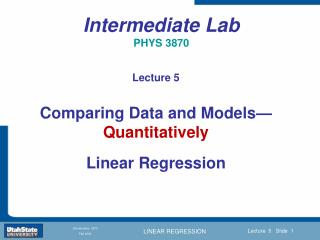DownloadDownload PresentationIntermediate Lab PHYS 3870

# Intermediate Lab PHYS 3870

Download Presentation## Intermediate Lab PHYS 3870

- - - - - - - - - - - - - - - - - - - - - - - - - - - E N D - - - - - - - - - - - - - - - - - - - - - - - - - - -
##### Presentation Transcript

1. Intermediate Lab PHYS 3870 Lecture 5 Comparing Data and Models—Quantitatively Linear Regression

2. Intermediate Lab PHYS 3870 Comparing Data and Models—Quantitatively Linear Regression References: Taylor Ch. 6, 7, 8 Also refer to “Glossary of Important Terms in Error Analysis”

3. Intermediate Lab PHYS 3870 Errors in Measurements and Models A Review of What We Know

4. Precision and Random (Statistical) Error

5. Standard Deviation

6. Standard Deviation of the Mean

7. Comparison with Other Data Percent Error = |X1 – X2| / ½(X1 + X2)

8. Direct Comparison with Standard Percent Error = |XMeasured – XStandard| / XStandard

9. Errors in Models—Error Propagation

10. Specific Rules for Error Propogation

11. General Formula for Multiple Variables

12. Intermediate Lab PHYS 3870 Rejecting Data Chauvenet’s Criterion

13. Chauvenet’s Criterion Data may be rejected if the expected number of measurements at least as deviant as the suspect measurement is less than 50%.

14. Central Probability of Gaussian Distribution

15. Chauvenet’s Criterion

16. Intermediate Lab PHYS 3870 Combining Data Sets Weighted Averages

17. Weighted Averages

18. Intermediate Lab PHYS 3870 Comparing Measurements to Models Qualitatively

19. What is Science? • The scientific method goes further in: • Developing a description (model) of the system behavior based on observation • Generalizing this description (model) to other behavior and other systems • That is to say, the scientific method is experimentation and modeling intertwined • It is the scientific method that distinguishes science from other forms of endeavor

20. Scientific Method: • Leads to new discoveries → how scientific progress is made! • Careful measurements, • Experiments • Models, Empirical • Laws,Generalization Hypothesis,Theory

21. Uncertainties in Observations Input Output SYSTEM The Universe • Observations characterize the system to within the uncertainty of the measurements • Uncertainties can arise from: • Limitations of instrumentation or measurement methods • Statistical fluctuations of the system • Inherent uncertainties of the system • - Quantum fluctuations • - Non-deterministic processes (e.g., chaos): • - There are systems where uncertainties dominate and preclude models predicting the outcome • - We will not (intentionally) deal with this type of system.

22. What is a Model?

23. What is a Model?

24. Intermediate Lab PHYS 3870 Dimensional Analysis

25. Units and Dimensions

26. Dimensional Analysis

27. Intermediate Lab PHYS 3870 Graphical Analysis

28. Graphical Analysis

29. Is it Linear?

30. Making It Linear

31. Linearizing Equations (1)

32. Linearizing Equations (2)

33. Special Graph Paper Linear Semilog Log-Log

34. Special Graph Paper Polar Linear

35. Magic Graph Paper

36. Intermediate Lab PHYS 3870 Comparing Measurements to Models Linear Regression